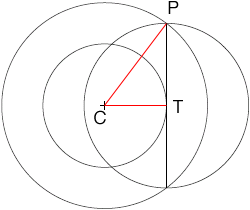SEARCH HOMEMath Central Quandaries & QueriesIn the diagram below, two concentric circles form an annulus. The vertical line is tangent to the inner circle, and forms the diameter of a third circle. Explain why the areas of the annulus and third circle are the same.Hi,

I added two red lines to your diagram and labeled three points.Each of the sides of the triangle PCT is a radius of one of the circles. What is the area of each of the circles? Triangle PCT is a right triangle(Why?). What does Pythagoras' theorem tell you about this triangle?

PennyMath Central is supported by the University of Regina and The Pacific Institute for the Mathematical Sciences.